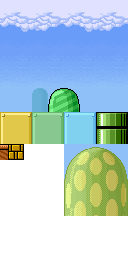# scroll

A short sketch showing the hardware's horizontal scrolling. The program scrolls an infinite randomly-generated background.

The source graphics for the background:Every 64 pixels, the function draw_column() draws an 8-character (64 pixel) wide column by copying rectangles from this source image into the character video RAM. This happens offscreen, a few characters to the right of the visible screen area.

This demo uses no sprites, and the backgrounds use only 157 of the available 256 characters. The whole demo uses only about 6K of Arduino flash, so there's plenty of scope for extension...

```#include <SPI.h>
#include <GD.h>

#include "platformer.h"

int atxy(int x, int y)
{
return (y << 6) + x;
}

// copy a (w,h) rectangle from the source image (x,y) into picture RAM
static void rect(unsigned int dst, byte x, byte y, byte w, byte h)
{
prog_uchar *src = platformer_pic + (16 * y) + x;
while (h--) {
GD.copy(dst, src, w);
dst += 64;
src += 16;
}
}

#define SINGLE(x, y) (pgm_read_byte_near(&platformer_pic[(y) * 16 + (x)]))

// Draw a random 8-character wide background column at picture RAM dst

void draw_column(unsigned int dst)
{
byte y;
byte x;
byte ch;

// Clouds and sky, 11 lines
rect(dst, 0, 0, 8, 11);

// bottom plain sky, lines 11-28
ch = SINGLE(0,11);
for (y = 11; y < 28; y++)
GD.fill(dst + (y << 6), ch, 8);

// randomly choose between background elements
byte what = random(256);
if (what < 10) {
// big mushroom thing
y = random(11, 18);
rect(dst + atxy(0, y), 8, 18, 8, 9);
y += 9;
byte i;
while (y < 28) {
rect(dst + atxy(0, y), 8, 23 + (i & 3), 8, 1);
i++, y++;
}
} else if (what < 32) {
// pair of green bollards
for (x = 0; x < 8; x += 4) {
y = random(20, 25);
rect(dst + atxy(x, y), 6, 11, 4, 3);
y += 3;
while (y < 28) {
rect(dst + atxy(x, y), 6, 13, 4, 1);
y++;
}
}
} else {
// hills
for (x = 0; x < 8; x += 2) {
y = random(20, 25);
rect(dst + atxy(x, y), 4, 11, 2, 3);
y += 3;
while (y < 28) {
rect(dst + atxy(x, y), 4, 13, 2, 1);
y++;
}
}
// foreground blocks
x = random(5);
y = random(11, 24);
byte blk = random(4);
rect(dst + atxy(x, y), blk * 4, 14, 4, 3);
y += 3;
while (y < 28) {
rect(dst + atxy(x, y), blk * 4, 17, 4, 1);
y++;
}
}

// Ground, line 28
ch = SINGLE(0,18);
GD.fill(dst + atxy(0,28), ch, 8);
// Underground, line 29
ch = SINGLE(0,19);
GD.fill(dst + atxy(0,29), ch, 8);
}

unsigned long xscroll;

void setup()
{
GD.begin();
GD.copy(RAM_CHR, platformer_chr, sizeof(platformer_chr));
GD.copy(RAM_PAL, platformer_pal, sizeof(platformer_pal));

int i;
for (i = 0; i < 256; i++)
GD.sprite(i, 400, 400, 0, 0, 0);

for (i = 0; i < 64; i += 8) {
draw_column(atxy(i, 0));
}
Serial.begin(1000000);
}

void loop()
{
xscroll++;
if ((xscroll & 63) == 0) {
// figure out where to draw the 64-pixel draw_column
// offscreen_pixel is the pixel x draw_column that is offscreen...
int offscreen_pixel = ((xscroll + (7 * 64)) & 511);
// offscreen_ch is the character address
byte offscreen_ch = (offscreen_pixel >> 3);
draw_column(atxy(offscreen_ch, 0));
}
GD.waitvblank();
GD.wr16(SCROLL_X, xscroll);
}
```